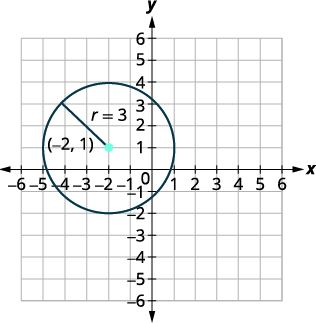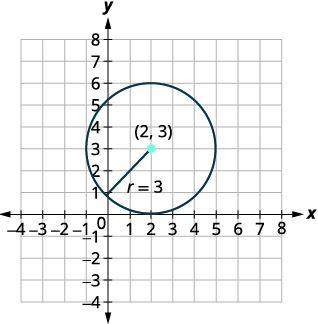## Section 1.2: Circles

### Learning Outcomes

• Write the equation of a circle in standard form
• Graph a circle

### DEFINITION OF A CIRCLE

A circle is all points in a plane that are a fixed distance from a given point in the plane.  The given point is called the center, $(h,k)$, and the fixed distance is called the radius, $r$, of the circle.### DERIVING THE STANDARD FORM OF A CIRCLE

To derive the equation of a circle, we can use the distance formula with the points $(h,k)$, $(x,y)$, and the distance $r$.

$d=\sqrt{(x_{2}-x_{1})^{2}+(y_{2}-y_{1})^{2}}$

Substitute the values.

$r=\sqrt{(x-h)^{2}+(y-k)^{2}}$

Square both sides.

$r^{2}=(x-h)^{2}+(y-k)^{2}$

### STANDARD FORM OF A CIRCLE

The standard form of a circle is as follows:

${\left(x-h\right)}^{2}+{\left(y-k\right)}^{2}=r^{2}$

## Write the Equation of a Circle in Standard Form

### Example 1: WRITE THE STANDARD FORM Equation OF A CIRCLE

Write the standard form of a circle with radius $3$ and center $(0,0)$.

Use the standard form of a circle.

${\left(x-h\right)}^{2}+{\left(y-k\right)}^{2}=r^{2}$

Substitute in the values $r=3, h=0, k=0$.

${\left(x-0\right)}^{2}+{\left(y-0\right)}^{2}=3^{2}$

Simplify.

${x}^{2}+{y}^{2}=9$

### Example 2: WRITE THE STANDARD FORM equation OF A CIRCLE

Write the standard form of a circle with radius $2$ and center $(-1,3)$.

### Example 3: finding the center and radius

Find the center and radius, then graph the circle: ${\left(x+2\right)}^{2}+{\left(y-1\right)}^{2}=9$.

Use the standard form of a circle.

${\left(x-h\right)}^{2}+{\left(y-k\right)}^{2}=r^{2}$

Identify the center $(h,k)$, and radius $r$.

${\left(x-(-2)\right)}^{2}+{\left(y-1\right)}^{2}=3^{2}$

The center is $(-2,1)$, and the radius is $3$.

Now graph the circle.  Plot the center first and then go up, down, left, and right 2 places.### Example 4: finding the center and radius

Find the center and radius, then graph the circle: ${4x}^{2}+{4y}^{2}=64$.

### GENERAL FORM OF A CIRCLE

The general form of a circle is as follows:

${x}^{2}+{y}^{2}+ax+by+c=0$

### Example 5: WRITE THE STANDARD FORM Equation OF A CIRCLE

Find the center and radius, then graph: ${x}^{2}+{y}^{2}-4x-6y+4=0$.

We need to rewrite this general form into standard form in order to find the center and radius.

${x}^{2}+{y}^{2}-4x-6y+4=0$

Group the x-terms and y-terms.  Collect the constants on the right right side.

${x}^{2}-4x+{y}^{2}-6y=-4$

Complete the squares.

${x}^{2}-4x+4+{y}^{2}-6y+9=-4+4+9$

Rewrite as binomial squares.

${\left(x-2\right)}^{2}+{\left(y-3\right)}^{2}=9$

The center is $(2,3)$, and the radius is $3$.

Now graph the circle.  Plot the center first and then go up, down, left, and right 3 places.### Example 6: WRITE THE STANDARD FORM Equation OF A CIRCLE

Find the center and radius, then graph: ${x}^{2}+{y}^{2}+8y=0$.

### Example 7: APPLYING THE DISTANCE AND MIDPOINT FORMULAS TO A CIRCLE EQUATION

The diameter of a circle has endpoints $(-1,-4)$ and $(7,2)$. Find the center and radius of the circle and also write its standard form equation.

The center of a circle is the center, or midpoint, of its diameter.  Thus the midpoint formula will yield the center point.

$M=\left(\frac{{x}_{1}+{x}_{2}}{2},\frac{{y}_{1}+{y}_{2}}{2}\right)$

$=\left(\frac{-1+7}{2},\frac{-4+2}{2}\right)$

$=\left(\frac{6}{2},\frac{-2}{2}\right)$

$=(3,-1)$

The center is $(3,-1)$.  The distance formula will be used to find the distance from the center to one of the points on the circle.  This will yield the radius:

$d=\sqrt{(x_{2}-x_{1})^{2}+(y_{2}-y_{1})^{2}}$

$d=\sqrt{(7-3)^{2}+(2-(-1))^{2}}$

$d=\sqrt{4^{2}+3^{2}}=5$

The distance from the center to a point on the circle is 5.  Therefore the radius is 5.  The center and radius can now be used to find the standard form of the circle:

Start with the standard form of a circle.

${\left(x-h\right)}^{2}+{\left(y-k\right)}^{2}=r^{2}$

Substitute in the values $r=5, h=3, k=-1$.

${\left(x-3\right)}^{2}+{\left(y-(-1)\right)}^{2}=5^{2}$

Simplify.

${\left(x-3\right)}^{2}+{\left(y+1\right)}^{2}=25$

### Example 8: Finding the Center of a Circle

The diameter of a circle has endpoints $\left(-1,-4\right)$ and $\left(5,-4\right)$. Find the center of the circle.

## Key Concepts

• A circle is all points in a plane that are a fixed distance from a given point on the plane.  The given point is called the center, and the fixed distance is called the radius.
• The standard form of the equation of a circle with center $(h,k)$ and radius $r$ is ${\left(x-h\right)}^{2}{+}{\left(y-k\right)}^{2}=r^{2}$

## Section 1.2 Homework Exercises

For the following exercises, write the standard form of the equation of the circle with the given radius and center $(0,0)$.

1. Radius: $7$

2. Radius: $9$

3. Radius: $\sqrt{2}$

4. Radius: $\sqrt{5}$

In the following exercises, write the standard form of the equation of the circle with the given radius and center.

5. Radius: $1$, center: $(3,5)$

6. Radius: $10$, center: $(-2,6)$

7. Radius: $2.5$, center: $(1.5,-3.5)$

8. Radius: $1.5$, center: $(-5.5,-6.5)$

For the following exercises, write the standard form of the equation of the circle with the given center and point on the circle.

9. Center: $(3,-2)$ with point $(3,6)$

10. Center: $(6,-6)$ with point $(2,-3)$

11. Center: $(4,4)$ with point $(2,2)$

12. Center: $(-5,6)$ with point $(-2,3)$

In the following exercises, find the center and radius and then graph each circle.

13. ${\left(x+5\right)}^{2}+{\left(y+3\right)}^{2}=1$

14. ${\left(x-2\right)}^{2}+{\left(y-3\right)}^{2}=9$

15. ${\left(x-4\right)}^{2}+{\left(y+2\right)}^{2}=16$

16. ${\left(x+2\right)}^{2}+{\left(y-5\right)}^{2}=4$

17. $x^{2}+{\left(y+2\right)}^{2}=25$

18. ${\left(x-1\right)}^{2}+{y}^{2}=36$

19. ${\left(x-1.5\right)}^{2}+{\left(y-2.5\right)}^{2}=0.25$

20. ${\left(x-1\right)}^{2}+{\left(y-3\right)}^{2}=\frac{9}{4}$

21. $x^{2}+y^{2}=64$

22. $x^{2}+y^{2}=49$

23. $2x^{2}+2y^{2}=8$

24. $6x^{2}+6y^{2}=216$

In the following exercises, identify the center and radius and graph.

25. $x^{2}+y^{2}+2x+6y+9=0$

26. $x^{2}+y^{2}-6x-8y=0$

27. $x^{2}+y^{2}-4x+10y-7=0$

28. $x^{2}+y^{2}+12x-14y+21=0$

29. $x^{2}+y^{2}+6y+5=0$

30. $x^{2}+y^{2}-10y=0$

31. $x^{2}+y^{2}+4x=0$

32. $x^{2}+y^{2}-14x+13=0$

33. Explain the relationship between the distance formula and the equation of a circle.

34. In your own words, state the definition of a circle.

35. In your own words, explain the steps you would take to change the general form of the equation of a circle to the standard form.